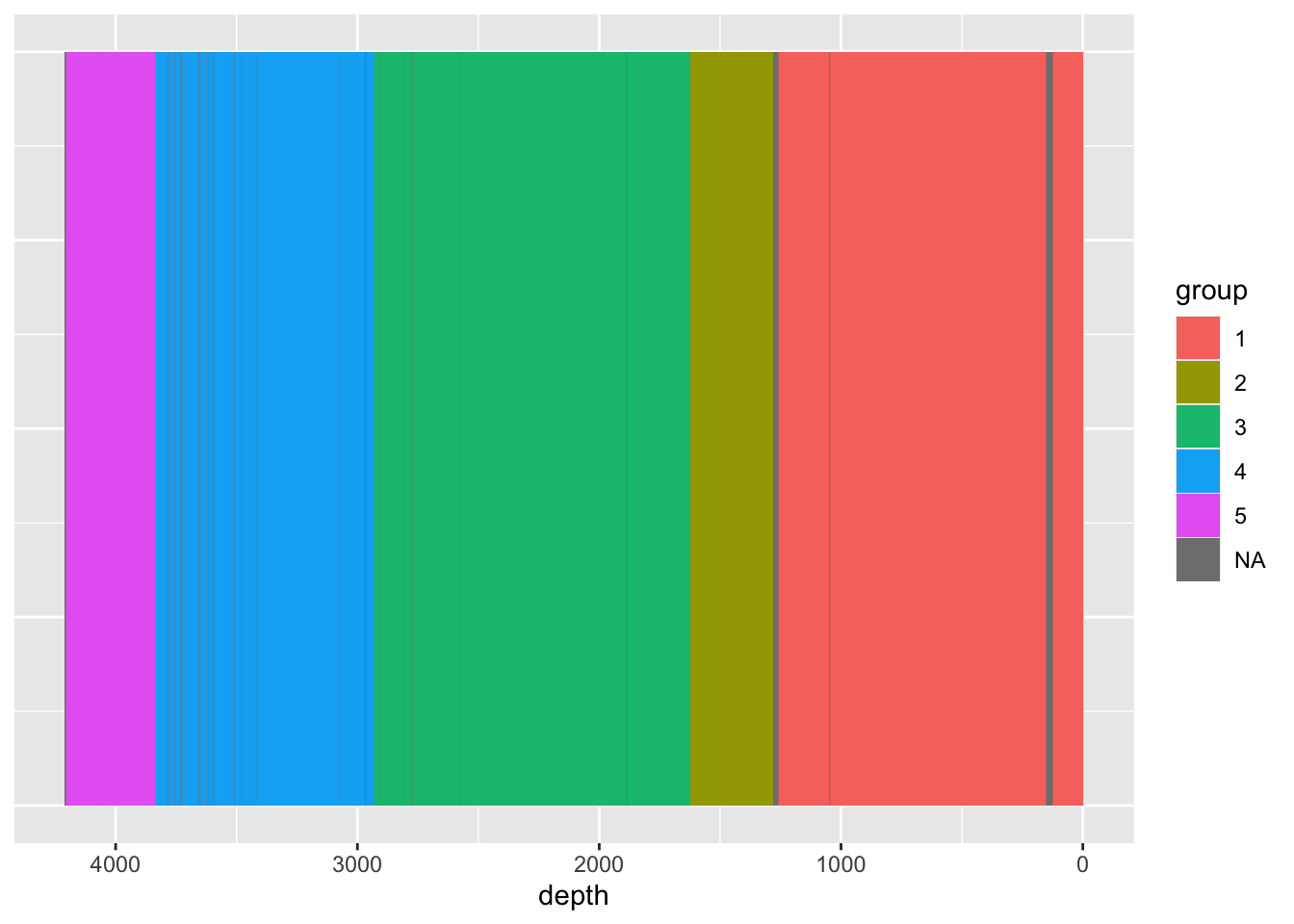## 6.5 Constrained Cluster Analysis

Constrained cluster analysis is helpful in identifying units of deposition in the sedimentary sequence. It forces each sample to remain in sequence, thus there are no recurring units along the stratigraphy.

``````bind_rows(tibble(depth = filter(CD166_19_xrf, qc == TRUE) %>% pull(depth),
group = rioja::chclust(dist(CD166_19_xrf_acomp)) %>%
cutree(k = 5) %>%
as.factor()),

CD166_19_xrf %>%
filter(qc == FALSE) %>%
mutate(group = NA) %>%
select(depth, group)) %>%
arrange(depth) %>%

ggplot() +
geom_tile(aes(x = depth, y = 1, fill = group)) +
scale_x_reverse() +
theme(axis.title.y = element_blank(),
axis.text.y = element_blank(),
axis.ticks.y = element_blank())``````